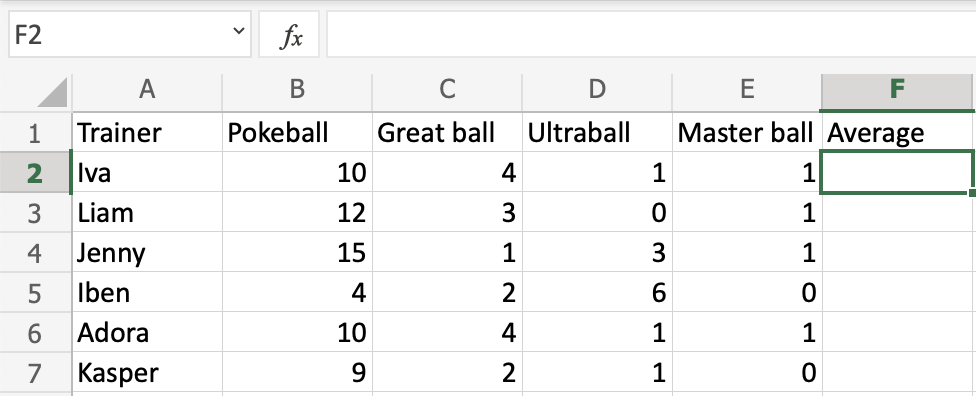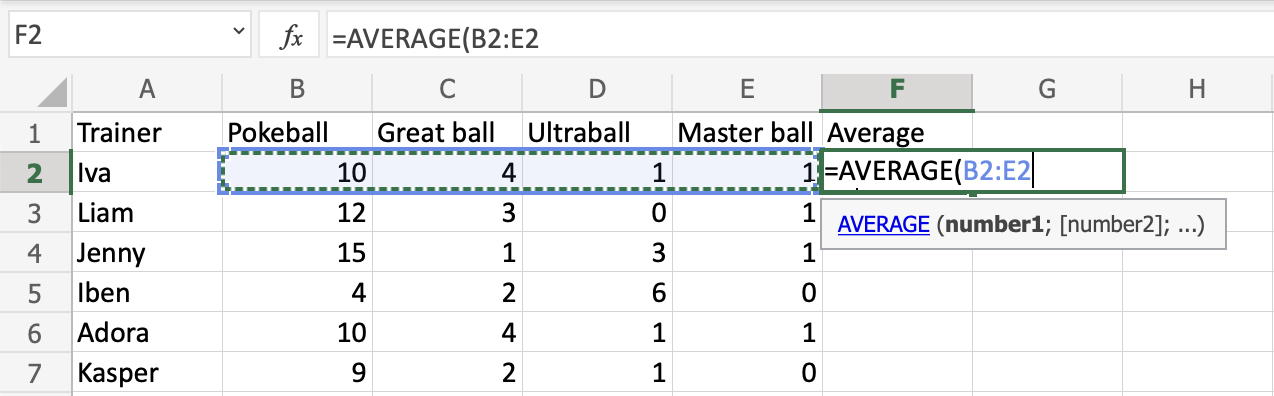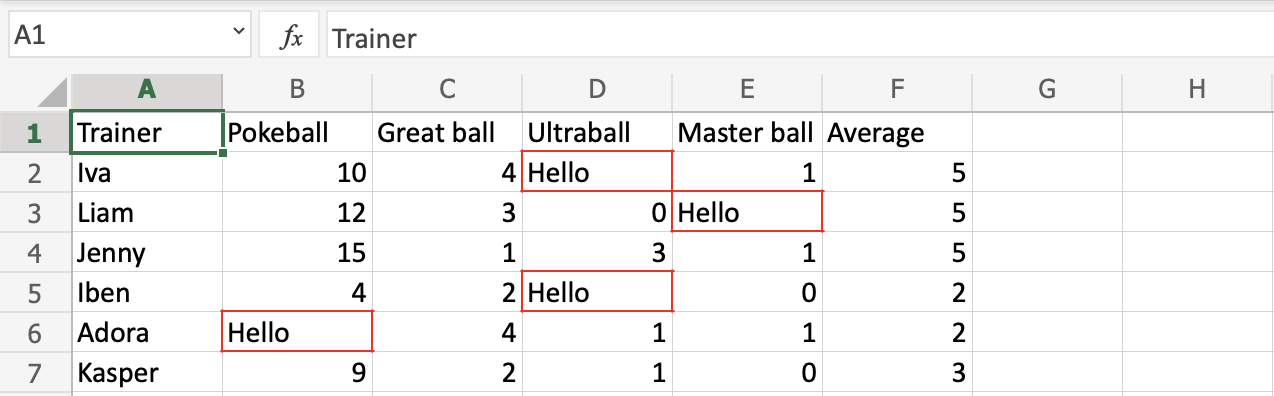# Excel AVERAGE Function

## AVERAGE Function

The AVERAGE function is a premade function in Excel, which calculates the average (arithmetic mean).

It is typed `=AVERAGE`

It adds the range and divides it by the number of observations.

Example:

The average of (2, 3, 4) is 3.
3 observations (2, 3 and 4)
The sum of the observations (2 + 3 + 4 = 9)
(9 / 3 = 3)
The average is 3

Note: There are multiple types of mean values. The most common type of mean is the arithmetic mean. You can learn more about the arithmetic mean here: Statistics Mean Tutorial.

Note: The AVERAGE function ignores cells with text.

Let's have a look at an example where we help the Pokemon trainers to calculate the average of Pokeballs per trainer.

How to use the `=AVERAGE` function, step by step:

1. Select a cell (`F2`)
2. Type `=AVERAGE`
3. Double click the AVERAGE command
4. Select a range (`B2:E2`)
5. Hit enter
6. Next, Fill `F2:F7`Copy ValuesGood job! You have successfully calculated the average using the AVERAGE function.

## Another Example

Example using cells with text:The function ignores cells with text and completes the calculation.